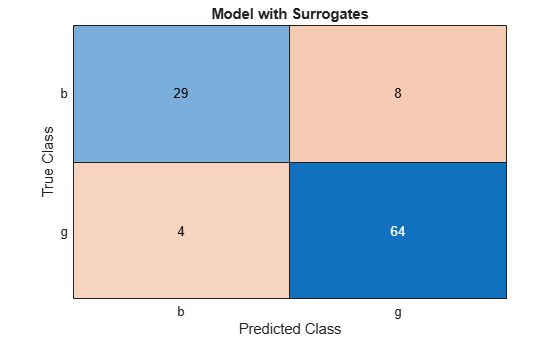Documentation

### This is machine translation

Mouseover text to see original. Click the button below to return to the English version of the page.

Note: This page has been translated by MathWorks. Click here to see
To view all translated materials including this page, select Country from the country navigator on the bottom of this page.

## Surrogate Splits

When the value of the optimal split predictor for an observation is missing, if you specify to use surrogate splits, the software sends the observation to the left or right child node using the best surrogate predictor. When you have missing data, trees and ensembles of trees with surrogate splits give better predictions. This example shows how to improve the accuracy of predictions for data with missing values by using decision trees with surrogate splits.

### Load Sample Data

Load the `ionosphere` data set.

`load ionosphere`

Partition the data set into training and test sets. Hold out 30% of the data for testing.

```rng('default') % For reproducibility cv = cvpartition(Y,'Holdout',0.3);```

Identify the training and testing data.

```Xtrain = X(training(cv),:); Ytrain = Y(training(cv)); Xtest = X(test(cv),:); Ytest = Y(test(cv));```

Suppose half of the values in the test set are missing. Set half of the values in the test set to `NaN`.

`Xtest(rand(size(Xtest))>0.5) = NaN;`

### Train Random Forest

Train a random forest of 150 classification trees without surrogate splits.

```templ = templateTree('Reproducible',true); % For reproducibility of random predictor selections Mdl = fitcensemble(Xtrain,Ytrain,'Method','Bag','NumLearningCycles',150,'Learners',templ);```

Create a decision tree template that uses surrogate splits. A tree using surrogate splits does not discard the entire observation when it includes missing data in some predictors.

`templS = templateTree('Surrogate','On','Reproducible',true);`

Train a random forest using the template `templS`.

`Mdls = fitcensemble(Xtrain,Ytrain,'Method','Bag','NumLearningCycles',150,'Learners',templS);`

### Test Accuracy

Test the accuracy of predictions with and without surrogate splits.

Predict responses and create confusion matrix charts using both approaches.

```Ytest_pred = predict(Mdl,Xtest); figure cm = confusionchart(Ytest,Ytest_pred); cm.Title = 'Model Without Surrogates';``````Ytest_preds = predict(Mdls,Xtest); figure cms = confusionchart(Ytest,Ytest_preds); cms.Title = 'Model with Surrogates';```All off-diagonal elements on the confusion matrix represent misclassified data. A good classifier yields a confusion matrix that looks dominantly diagonal. In this case, the classification error is lower for the model trained with surrogate splits.

Estimate cumulative classification errors. Specify `'Mode','Cumulative'` when estimating classification errors by using the `loss` function. The `loss` function returns a vector in which element `J` indicates the error using the first `J` learners.

```figure plot(loss(Mdl,Xtest,Ytest,'Mode','Cumulative')) hold on plot(loss(Mdls,Xtest,Ytest,'Mode','Cumulative'),'r--') legend('Trees without surrogate splits','Trees with surrogate splits') xlabel('Number of trees') ylabel('Test classification error')```The error value decreases as the number of trees increases, which indicates good performance. The classification error is lower for the model trained with surrogate splits.

Check the statistical significance of the difference in results with by using `compareHoldout`. This function uses the McNemar test.

`[~,p] = compareHoldout(Mdls,Mdl,Xtest,Xtest,Ytest,'Alternative','greater')`
```p = 0.1051 ```

The low p-value indicates that the ensemble with surrogate splits is better in a statistically significant manner.

### Estimate Predictor Importance

Predictor importance estimates can vary depending on whether or not a tree uses surrogate splits. After estimating predictor importance, you can exclude unimportant predictors and train a model again. Eliminating unimportant predictors saves time and memory for predictions, and makes predictions easier to understand.

Estimate predictor importance measures by permuting out-of-bag observations. Then, find the five most important predictors.

```imp = oobPermutedPredictorImportance(Mdl); [~,ind] = maxk(imp,5)```
```ind = 1×5 3 5 27 7 8 ```
```imps = oobPermutedPredictorImportance(Mdls); [~,inds] = maxk(imps,5)```
```inds = 1×5 5 3 7 27 8 ```

The five most important predictors are the same, but the orders of importance are different.

If the training data includes many predictors and you want to analyze predictor importance, then specify `'NumVariablesToSample'` of the `templateTree` function as `'all'` for the tree learners of the ensemble. Otherwise, the software might not select some predictors, underestimating their importance. For an example, see Select Predictors for Random Forests.

Download ebook